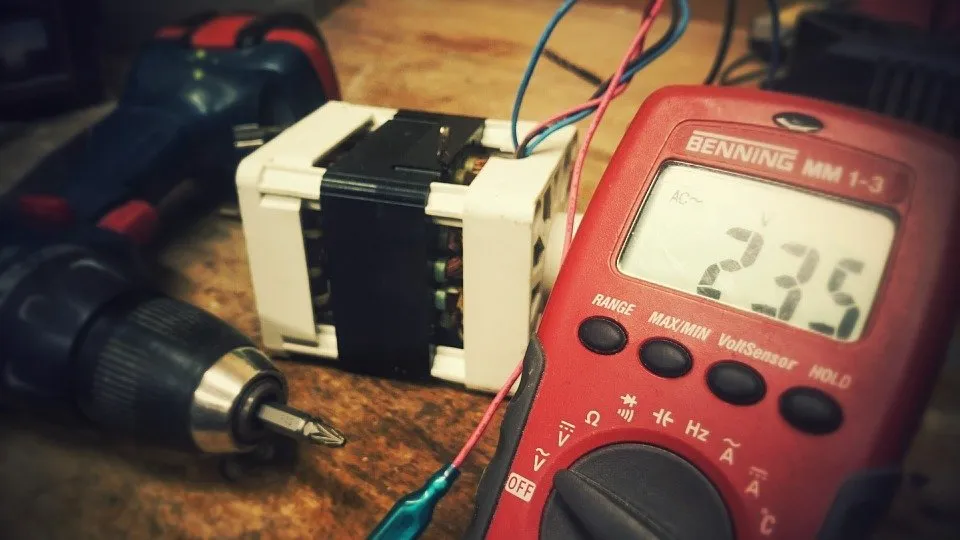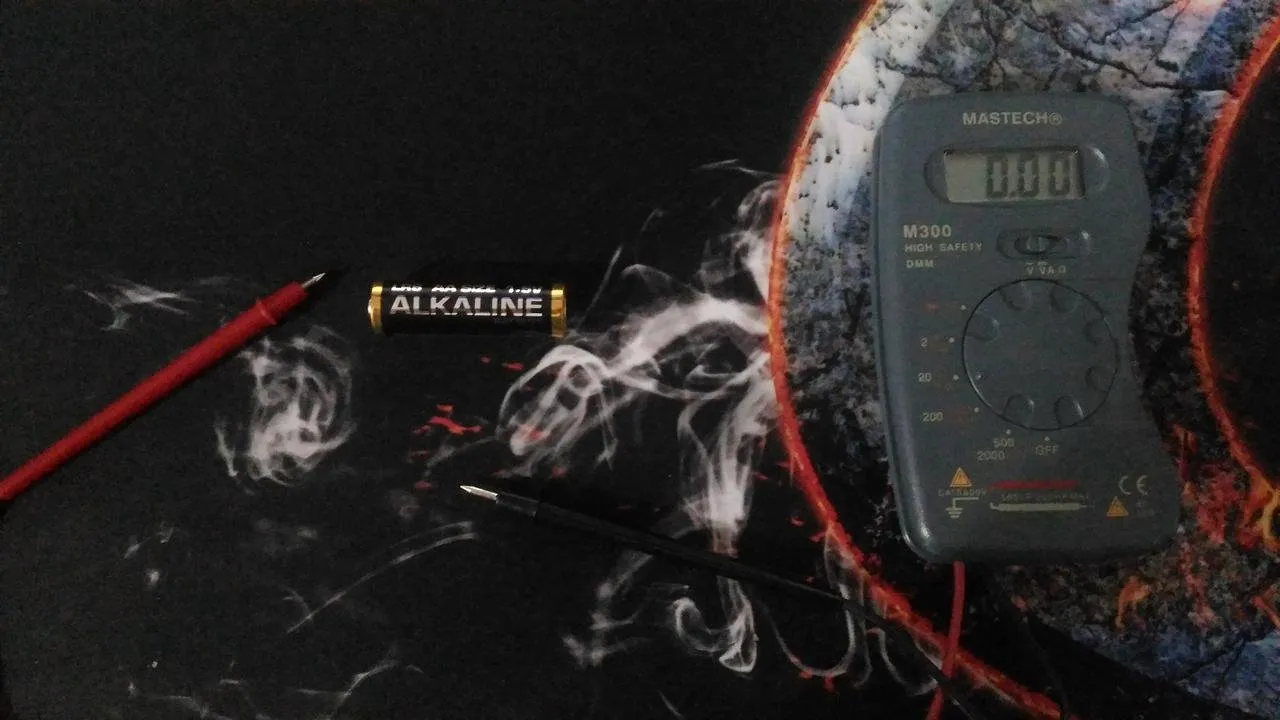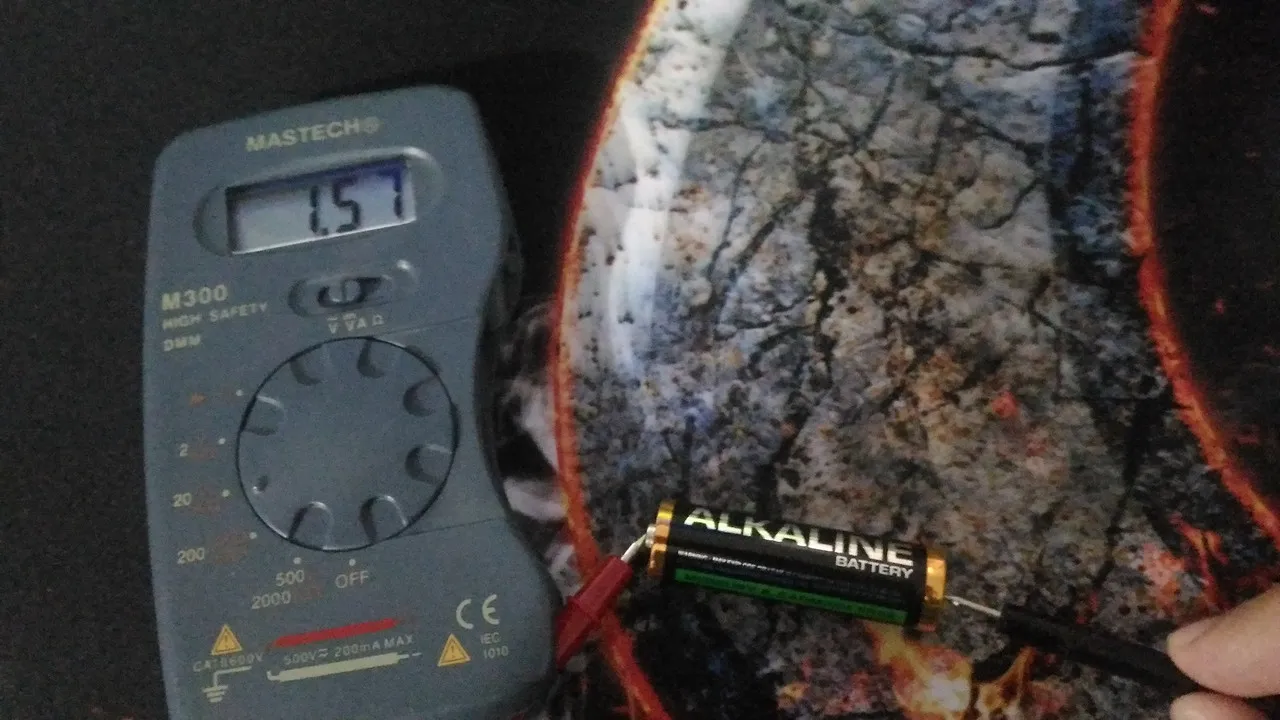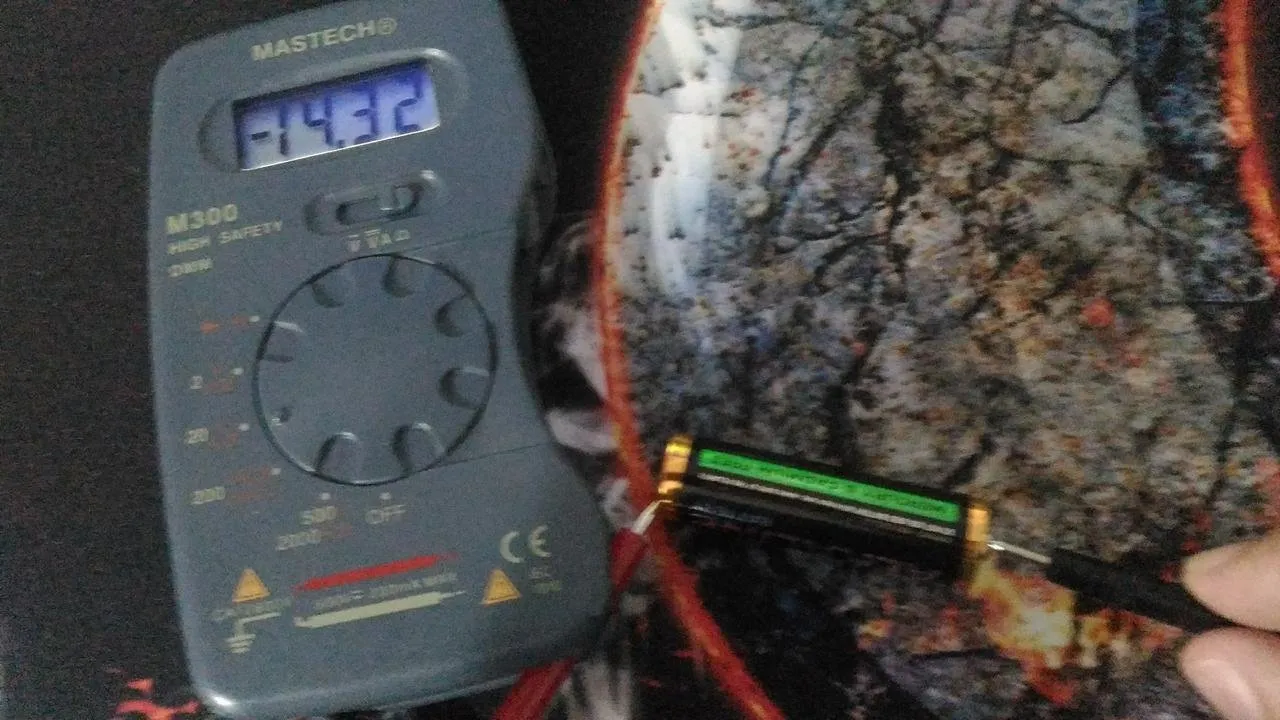# Physics - Electromagnetism - Electrical measuring instrumentsImage source: https://www.maxpixel.net/

## Introduction

Hello it's a me again Drifter Programming! Today we continue with Electromagnetism to get into how we measure the different metrics and units of electronic circuits, where electrical measuring instruments or devices come into play. If you don't know about those metrics already this post will not make so much sense and so I suggest you to know about: electric current, potential (voltage), capacitance, resistance, power etc. already. So, without further do, let's get straight into it!

## What are electrical measuring instruments?

Electrical measuring instruments are devices used for measuring electrical aspects and quantities such as current, voltage, resistance and power. It's important to measure them to determine if the electrical system has faults or not. For example when having problems with electornic equipment we can detect the problem using some instrument which is called a meter and afterwards fix it.

Such devices have the following three functions:

1. Indicating Function -> They provide information about the quantity they are measuring.
2. Recording Function -> They output/record what they measure.
3. Controlling Function -> They control processes.

And also have some characteristics which are static or dynamic:

• Static characteristics are the accurancy, sensitivity and reproducibility.
• Dynamic characteristics are related to rapidly changing quantities and so are about the dynamic relations of the input and output.

## What types do we have?

We can split them up based on their usage which can be:

1. Detecting and indicating instruments (ammeter, galvanometer, voltmeter etc.)
2. Recorders (continuous line recorder, dotted line recorder, event recorder etc.)
3. Transducers (voltage transducer, current transducer, active power transducer etc.)
4. Stabilized power supplies (constant voltage/current power supply)
5. Oscilloscopes (measuring oscilloscope, observation oscilloscope etc.)
6. Energy meters (watt-hour meter, var-hour meter, static meter etc.)
7. Signal generators (amplitude or frequency modulated signal generator)
8. Measuring bridges (Wheatstone, Thompson and transformer bridge)
9. Accessories (series resistor,  series capacitor, instrument lead, probe etc.)

And we can also split them out depending on if they are:

• Absolute (or Primary) instruments -> Which give us the magnitude/value of the quantity without the need of any other instrument for comparison.
• Secondary instruments -> Which have a deflection in the magnitude and need to be calibrated and so their measurements have to be compared with another standard intrument before using them.

Another way is depending on how they produce the results:

• Deflection type -> Such an instrument has a pointer which deflects from its initial position and the actual deflection is the value of the quantity measured.
• Null type -> Such an instrument has a stationary pointer and maintains this position using a restorance force which can then be translated into the quantity.

Let's get into what exactly some of they are doing:

• Ammeter (Ampermeter) -> Measures current
• Capacitance meter -> Measures the capacitance of a component
• Cos Phi meter -> Measures the power factor
• Electricity meter -> Measures the energy dissipation
• ESR meter -> Measures the equivalent series resistance of capacitors
• LCR meter -> Measures the inductance, capacitance and resistance of a component
• Ohmmeter -> Measures the resistance of a component
• Oscilloscope ->  Displays waveform of a signal, allows measurement of frequency, timing, peak excursion, offset, ...
• Signal generator -> Generates signals for testing purposes
• Wattmeter -> Measures the power consumption
• Voltmeter ->  Measures the potential difference between two points in a circuit

## Getting into some more in-depth

Let's get into some of the most essential ones more in-depth.

### Ammeter:

Ammeters are instruments that measure the current in a circuit. The flow of current is measured in amps or Ampere (A). There are various designs and sizes that are used depending on the application and magnitude of current. We can use them to check if current flows through a wire and so if the wiring has be done correctly in a bulding. We can also use them to find malfunctions, short circuits etc. in electrical equipment. An ideal ammeter is a two terminal device which is polarized. It connects in series with the circuit element to measure the sign and magnitude of the current that flows through that component. In electronic circuits we note it as a circle with an 'A' in the middle.

There are many types of ammeters depending on their structure:

• The D'Arsonval galvanometer is a moving coil ammeter which uses magnetic deflections to calculate the  current that passes through a coil placed in a magnetic field.
• Moving magnet ammeters which are similar to moving-coil ones, but can carry larger currents, because they have a permanent magnet moving the needele.
• Electrodynamic ammeters, which use an electromagnet and so can calculate both alternating and direct current.
• Moving iron ammeters, which use a piece of iron which moves by the electromagnetic force of a fixed coil of wire.
• Hot-wire ammeters, which expand as the current passing by causes heat dissipation.
• Digital ammeters, which produce a shunt resistor and produce a calibrated voltage proportional to the current.
• Integrating ammeters, which sum of the current over time and so give the current as the result of current and time.

### Voltmeter:

Voltmeters are devices that measure the voltage or potential difference between two points of a circuit. The voltage is measured in Volts (V). They are connected in parallel to the circuit and have various styles. Analog ones move a pointer across a scale in proportion to the circuit, while digital ones give a numerical display of voltage. To calculate the potential difference between two points of the circuit we use the two terminals of the voltmeter and connect them to the circuit at these exact points (in parallel). In electronic circuits a volt meter is represented by a circle with a 'V' inside.

### Ohmmeter:

Ohmmeters are devices that measure the electrical resistance through a circuit in the unit of measurent which is called Ohm (Ω). We use them in installations where the correct resistance is important for the proper functioning of an electronic device. They can mostly also check if the current flow is continuous or not. Ohmmeters can be analog or digitial and the digital ones have a much better accurancy and are also much easier to read. In electronic circuits we note them with a circle that has a 'Ω' inside.

### Multimeter:

A multimeter is a popular, versatile, general purpose instrument that measures voltage, current, resistance and sometimes also other quantities. This device combines several measurement function in one single device/unit and is mostly hand-held, which makes it easy to carry around and useful for basic fault finding and field service work.

A multimeter mostly has:

• a display which shows the measurement in usually four digits and also with negative sign
• a selection knob that allows us to set the multimeter to read a specific quantity AND
• ports which are used for grounding and measuring large currents, voltages etc.

Two probes which are black and red are plugged in the front ports, where the black one represents the '-' and the red one the '+' and so when calculating anything we should always remember putting them in the right side.

## An Application

I have a multimeter at home and so I thought, why not make a small measurement? I calculate the voltage of a 1.5V battery and also it's resistance by just connecting the terminals to the poles of the battery. For the voltage I do it in the right order, for the resistance in the "wrong" so that the result comes out negative!

The battery and multimeter:Measuring the voltage:We get a result of 1.57V, which is not far away of the 1.5V that the battery is noted to be!

Measuring the resistance:We get a resistance of -14.32kΩ, because of "wrong" placement.

## REFERENCES:

### Electric fields:

Getting into Electromagnetism -> electromagnetim, electric charge, conductors, insulators, quantization

Coulomb's law with examples -> Coulomb's law, superposition principle, Coulomb constant, how to solve problems, examples

Electric fields and field lines -> Electric fields, Solving problems around Electric fields and field lines

Electric dipoles -> Electric dipole, torque, potential and field

Electric charge and field Exercises -> examples in electric charges and fields

### Electric flux:

Electric flux and Gauss's law -> Electric flux, Gauss's law

Applications of Gauss's law (part 1) -> applying Gauss's law, Gauss applications

Applications of Gauss's law (part 2) -> more Gauss applications

Electric flux exercises -> examples in electric flux and Gauss's law

### Electric potential:

Electric potential energy -> explanation of work-energy, electric potential energy

Calculating electric potentials -> more stuff about potential energy, potential, calculating potentials

Millikan's Oil Drop Experiment -> Millikan's experiment, electronvolt

Cathode ray tubes explained using electric potential -> cathode ray tube explanation

Electric potential exercises (part 1) -> applications of potential

Electric potential exercises (part 2) -> applications of potential gradient, advanced examples

### Capacitance:

Capacitors (Condensers) and Capacitance -> Capacitors, capacitance, calculating capacitance

How to solve problems around Capacitors -> combination, solving problems, simple example

Electric field energy and density -> Electric field energy, energy density

Dielectric materials -> Dielectrics, dielectric constant, permittivity and strength, how to solve problems

Electric capacitance exercises -> examples in capacitance, energy density and dielectrics

### Current, resistance and EMF:

Electric current -> Electric current, current density

Electrical resistivity and conductivity -> Electrical resistivity, conductivity, thermal coefficient of resistivity, hyperconductivity

Electric resistance -> Resistance, temperature, resistors

Electromotive Force (EMF) and Internal resistance -> Electromotive force, internal resistance

Power and Wattage of Electronic Circuits -> Power in general, power/wattage of electronic circuits

Electric current, resistance and emf exercises -> exampes in all those topics

### Direct current (DC) circuits:

Resistor Combinations -> Resistor combinations, how to solve problems

Kirchhoff's laws with applications -> Kirchhoff's laws, how to solve problems, applications

And this is actually it for today's post and I hope that you enjoyed it!

Next time we will get into combinational electronic circuits that contain resistors and capacitors, which are the so called R-C circuits.

C ya!

H2
H3
H4
3 columns
2 columns
1 column
1 Comment